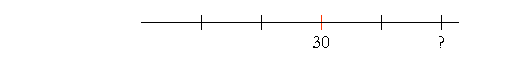S k i l l
i n
A R I T H M E T I C

Lesson 29  Section 3

# To find the Base the number that follows "of"

Back to Section 1

The general rule

Example 1.   7 is 25% of what number?

Here, the Base is missing, the number that follows of.  This is an elementary problem in finding the Base. It's Example 11 in Lesson 28.

 Example 2.   14 is 66 23 % of what number? (See Lesson 16.)

14 is two thirds of what number?But if 14 is two thirds, then half of 14 is one third.  Half of 14 is 7.  And 7 is one third -- of 21.14 is 66 23 % of 21.

Any number will be two thirds of one and a half times itself.

Solution 2.   If 14 is two thirds of some number, then proportionally:

As 2 is to 3, then 14 is to ?

2 : 3 = 14 : ?

"2 goes into 14 seven times. Seven times 3 is 21."

2 : 3 = 14 : 21.

14 is two thirds of 21.

Compare Lesson 18, Examples 5 and 6.

Example 3.   30 is 60% -- three fifths -- of what number?

Solution 1.  If 30 is three fifths of some number,then a third of 30 is one fifth.A third of 30 is 10.  And 10 is one fifth -- of 50.

30 is 60% of 50.

Solution 2.   If 30 is three fifths of some number, then proportionally:

3 : 5 = 30 : ?

To produce 30,  3 has been multiplied by 10. Therefore 5 must also be mutiplied by 10.

3 : 5 = 30 : 50.

30 is three fifths -- 60% -- of 50.

Example 4.   27 is 75% -- three fourths -- of what number?

Solution.  Proportionally:

3 : 4 = 27 : ?

"3 goes into 27 nine times. Nine times 4 is 36.

3 : 4 = 27 : 36..

27 is three fourths -- 75% -- of 36.

It turns out that we will always be able to find the Base by the method of proportions, which is an instance of The Rule of Three.

 As the Percent is to 100 so the Amount is to the Base. P : 100 = A : B.

Example 5.   \$36 is 4% of how much?

 Solution. 4 : 100 = \$36 : ?

"4 goes into 36 nine times. Nine times 100 is 900."

4 : 100 = \$36 : \$900.

\$36 is 4% of \$900.

 Solution. 6 : 100 = 42 : ?

42 is seven times 6.  Therefore, the missing term must be seven times 100.

6 : 100 = 42 : 700.

42 is 6% of 700.

Here is the check:

1% of 700 is 7.  Therefore, 6% is 6 × 7 = 42.

Example 7.   8% of what number is 20?

 Solution. 8 : 100 = 20 : ?

On dividing both terms on the left by 4

2 : 25 = 20 : ?

"2 goes into 20 ten times. Ten times 25 is 250.

2 : 25 = 8 : 100 = 20 : 250.

20 is 8% of 250.

Check:  1% of 250 = 2.5

 Therefore, 8% of 250 = 8 × 2.5 = 2.5 × 8  (Two and a half times 8) (Lesson 27, Examples 10 and 11) = 20.

Example 8.   9 is 15% of what number?

 Solution. 15 : 100 =9 : ?

On dividing by 5:

3 : 20 = 9 : ?

3 has been multiplied by 3.  Therefore, 20 must be multiplied by 3:

3 : 20 =9 : 60.

9 is 15 % of 60.

(10% of 60 is 6.  5% is 3.  6 + 3 = 9.)

Example 9.   24 is 150% of what number?

 Solution. 150 : 100 =24 : ?

On dividing by 50:

3 : 2 = 24 : ?

"3 goes into 24 eight times. Eight times 2 is 16."

3 : 2 =24 : 16.

24 is 150 % of 16.

Check: 24 is 150% of -- 1½ times -- 16.  (16 + 8 = 24.)

(Lesson 16.)

Example 10.   Maria is retired and withdraws money from her retirement account. But a tax of 20% is automatically withheld. If she needs \$1200, how much must she actually request?

Solution 1..   Since 20% will be withheld, Maria will receive 80% of her request.  So the question is:  \$1200 is 80% of how much?

80 : 100 = 4 : 5 = 1200 : ?

4 has been multiplied by 300. Therefore, 5 must be multiplied by 300:

4 : 5 =1200 : 1500.

\$1200 is 80% of \$1500.

In other words, since 5 is one and a quarter times 4, then Maria must always request one quarter more than what she needs.  One quarter of \$1200 is \$300. Therefore she must request \$1200 + \$300 = \$1500.

It is then a simple matter to see that 20%, or a fifth, of \$1500 is \$300, so that her net amount will in fact be \$1200.

Solution 2.   We have seen that to find the Amount, we multiply.

Amount = Base × Percent

Therefore, according to the relationship between multiplication and division, to find the Base, we divide.

Base = Amount ÷ Percent

In this Example, we are to divide 1200 by 80%, or 4/5.  But we have seen that division is multiplication by the reciprocal. That will result in multiplying by 5/4 or 1¼.

1¼ × \$1200 = \$1200 + \$300 =\$1500.

Example 12.   Michelle paid \$82.68 for a pair of shoes -- but that included a tax of 6%.  What was the actual price of the shoes before the tax?

Solution.  This is Example 7:  Percent with a Calculator.

Please "turn" the page and do some Problems.

or

Continue on to the next Lesson.

Section 1 of this Lesson